# OECD Purchasing power parities (PPP) data analysis 5 - PCA (Principal Component Analysis) using R.Photo by Aron Visuals on Unsplash

This post is following of the above post.
In this post, I will do PCA(Principal Component Analysis).

I refer below web site.
Principal Component Analysis (PCA) 101, using R | by Peter Nistrup | Towards Data Science

Firstly, I will make subset for PCA from df.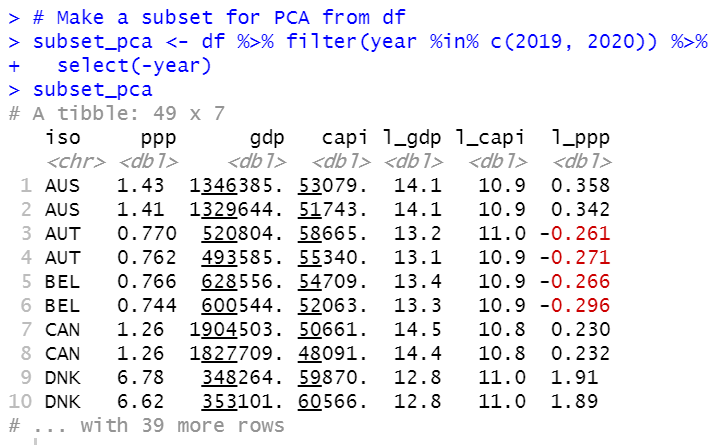Now, I have subset_pca data frame which has 6 mumerical varibles, ppp, gdp, capi, l_gdp, l_capi and l_ppp.

Then, I use prcomp() function for PCA.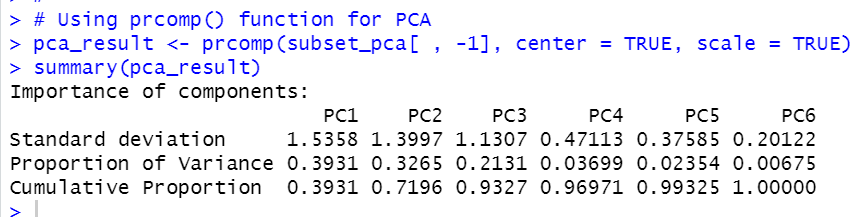Above results tells that PC1 counts 39% of variables, PC2 counts 32% variable. So, PC1 and PC2 counts 72% variable.

Then, I make a plot to visualize PCA result using screeplot() function.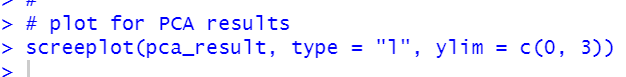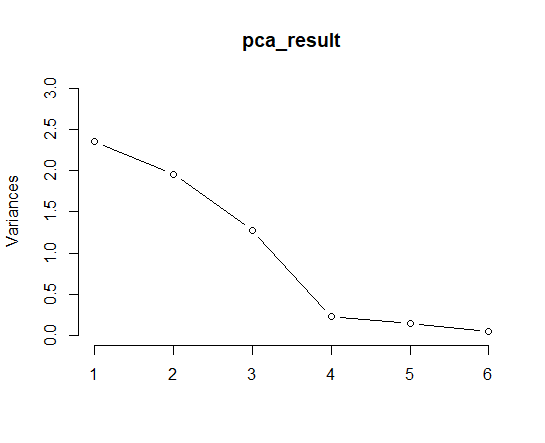Let's make PC1 vs PC2 plot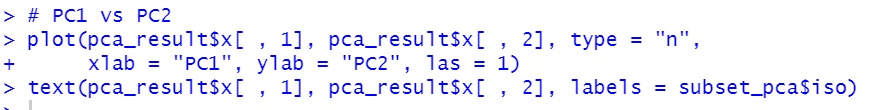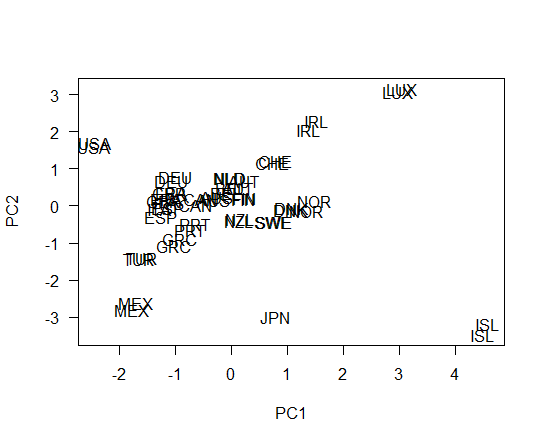Then, I add rownames to pca_result\$x.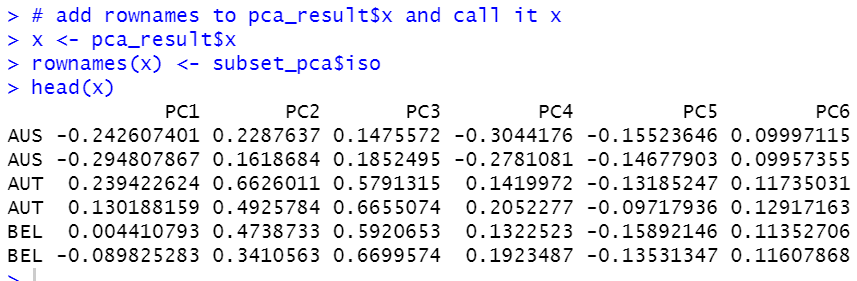Then, let's make a hierarchial culstering dendrogram.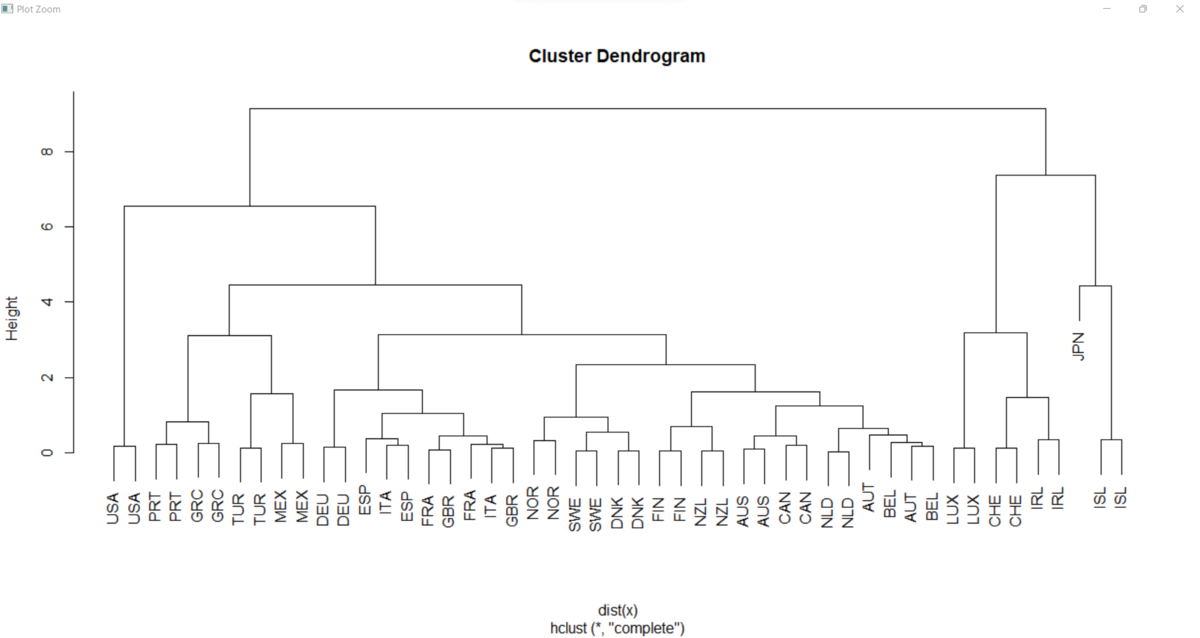That's it. Thank you!.

The next post is